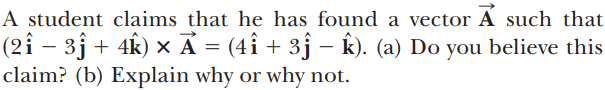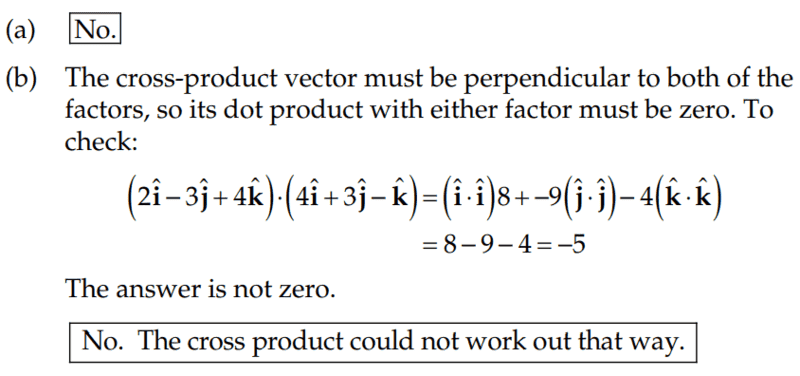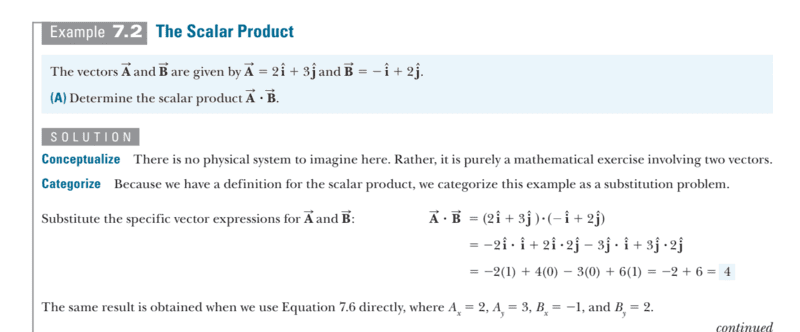# Cross product for two vectors

Callumnc1
Homework Statement:
Trying to find whether there is a vector that can solve the cross product between two vectors.
Relevant Equations:
Cross product formula: A cross B = ABcos(theta)
Dot product formula: A dot B = ABsin(theta)
Hi!

For this problem,The solution is,However, I don't understand their solution at all. Can somebody please explain their reasoning in more detail.

Many thanks!

Homework Helper
Gold Member
If ##\mathbf{B} \times \mathbf{A} = \mathbf{C}##, what is the angle between ## \mathbf{B}## and ## \mathbf{C}## (assuming ##\mathbf{C} \neq 0##)?

•Callumnc1 and topsquark
Callumnc1
Thank for your reply! 90 degrees I think because the cross product of two vector is always perpendicular to the plane formed by the two vectors. Many thanks!

Callumnc1
Thanks, I see now, so since B and C must be at 90 degrees for it to be a cross product then we take the scalar product to find whether there is any component of B on C or C on B. And the scalar product of all components must be zero if B and C are to be perpendicular. Do you please know what we call it when the B and C are not 90 degrees to each other?

Many thanks!

Homework Helper
Gold Member
2022 Award
Cross product formula: A cross B = ABcos(theta)
Dot product formula: A dot B = ABsin(theta)
Assuming θ is the angle between the vectors, you have that, ahem, crossed.
##||\vec A\times\vec B||=||\vec A||.||\vec B||.| \sin(\theta)|##
##||\vec A.\vec B||=||\vec A||.||\vec B||.| \cos(\theta)|##.

the scalar product of all components must be zero
That wording suggests a misunderstanding. The scalar product of two vectors is a single number. There is not a separate scalar product for each component.

what we call it when the B and C are not 90 degrees to each other?
Do you mean for two vectors in general? Maybe "non orthogonal"? Not aware of anything more concise.

•Callumnc1
Callumnc1
Assuming θ is the angle between the vectors, you have that, ahem, crossed.
##||\vec A\times\vec B||=||\vec A||.||\vec B||.| \sin(\theta)|##
##||\vec A.\vec B||=||\vec A||.||\vec B||.| \cos(\theta)|##.

That wording suggests a misunderstanding. The scalar product of two vectors is a single number. There is not a separate scalar product for each component.

Do you mean for two vectors in general? Maybe "non orthogonal"? Not aware of anything more concise.
Thanks for your reply! Sorry, what did you please mean when you said that we cannot take the scalar product of each component. My textbooks shows:Many thanks!

Callumnc1
Do you mean for two vectors in general? Maybe "non orthogonal"? Not aware of anything more concise.
Yeah, thanks I mean for any two vectors in general. I think cross product is orthogonal. Many thanks!

Homework Helper
Gold Member
2022 Award
what did you please mean when you said that we cannot take the scalar product of each component
Sorry, I didn’t manage to express that quite correctly.
Your remark in post #4 implies you think that if ##\vec x=x_i\vec i+x_j\vec j+x_k\vec k## and ##\vec y=y_i\vec i+y_j\vec j+y_k\vec k## are orthogonal then each of the scalar products ##x_i\vec i.y_i\vec i##, ##x_j\vec j.y_j\vec j##, etc., must be zero. My point is that the scalar product ##\vec x.\vec y## is the sum of those individual products, and it is only that sum that needs to be zero.

•Callumnc1
Callumnc1

If vectors x and y are not orthogonal, then we can't call it a cross product since the vectors are not at right angles. Is there a specific term used for non-orthogonal vectors?

Many thanks!

Homework Helper
Gold Member
2022 Award
Is there a specific term used for non-orthogonal vectors?
Not that I am aware of.

•Callumnc1
Callumnc1
Not that I am aware of.
Ok thank you!

Staff Emeritus
$$\vec A \cdot \vec B = \|\vec A\| \|\vec B\| \cos\theta$$
•